## Is the equation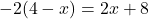an identity? Explain your reasoning. A. Yes, because solving the equation gives a s

Question

Is the equationan identity? Explain your reasoning.

A. Yes, because solving the equation gives a statement that is never true.

B. Yes, because solving the equation gives a statement that is always true.

C. No, because solving the equation gives a statement that is never true.

D. No, because solving the equation gives a statement that is always true.

help I don’t know what an identity is or how to explain

in progress 0
5 months 2021-08-13T02:09:42+00:00 1 Answers 4 views 0

1. Given:

The equation is.

To find:

Whether the given equation is an identity or not.

Solution:

If both sides of an equation are equal for all values of variables, then it is called identity.

We have,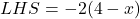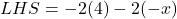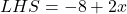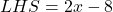Now,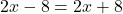On subtracting 2x from both sides, we get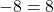This statement is never true.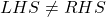So, the given equation is not an identity because solving the equation gives a statement that is never true.

Therefore, the correct option is C.